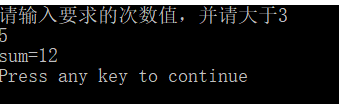• 斐波那切数列 【问题描述】 斐波那切数列0，1，1，2，3，5，8，13，21，34，55……从第三项起，每一项都是紧挨着的前两项的和。写出计算斐波那切数列的任意一个数据项递归程序。 【输入格式】 输入所求的项数。...


斐波那切数列
【问题描述】
斐波那切数列0，1，1，2，3，5，8，13，21，34，55……从第三项起，每一项都是紧挨着的前两项的和。写出计算斐波那切数列的任意一个数据项递归程序。
【输入格式】
输入所求的项数。
【输出格式】
输出数据项的值。
【输入样例】fbi.in
10
【输出样例】fbi.out
34

#include<iostream>
#include<cstdio>
#include<algorithm>
#include<cstring>
#include<string>
using namespace std;
int dg(int m)
{
if(m==1)return 0;
if(m==2)return 1;
else return dg(m-1)+dg(m-2);
}
int main()
{
int m;
cin>>m;
cout<<dg(m);
return 0;
}

转载于:https://www.cnblogs.com/sssy/p/6601953.html
展开全文• C语言斐波那切数列求和 ** 斐波那切数列数列是：0,1,1,2,3,5,8,13… 其中a0=0，a1=1，a2=1… 通项为：an=a(n-1)+a(n-2) (n>=3)，即后一项为前两项之和 具体代码为 #include<stdio.h> void main() { int a,...
**
C语言斐波那契数列求和
**
斐波那契数列，又称黄金分割数列，指的是这样一个数列：0、1、1、2、3、5、8、13、21、34、……在数学上，斐波纳契数列以如下被以递归的方法定义：F（0）=0，F（1）=1，F（n）=F(n-1)+F(n-2)（n≥2，n∈N*）在现代物理、准晶体结构、化学等领域，斐波纳契数列都有直接的应用，为此，美国数学会从1963起出版了以《斐波纳契数列季刊》为名的一份数学杂志，用于专门刊载这方面的研究成果。
也就是说：
斐波那契数列是：0,1,1,2,3,5,8,13…
其中a0=0，a1=1，a2=1…
通项为：an=a(n-1)+a(n-2)     (n>=3)，即后一项为前两项之和
具体代码为
#include<stdio.h>
void main()
{
int a,b,c,count,i=3,sum=0;
a=1;
b=1;
c=a+b;
puts("请输入要求的次数值，并请大于3");
scanf("%d",&count);
sum=a+b+c;
while(i<count){
a=b;
b=c;
c=a+b;
sum+=c;
i++;
}
printf("sum=%d\n",sum);
}

输入5，结果如下图所示：展开全文c语言 程序设计
• python实现斐波那切数列，直观明了直接可以运行，有助于学习和思考
• //斐波那切数列的递归算法 template <typename Type> Type fibonacci_1(int n,Type flag){ if(n<=1)return 1; return fibonacci_1(n-1,flag)+fibonacci_1(n-2,flag); } 递归免重复计算算法 //斐波那切...
递归普通算法
//斐波那切数列的递归算法
template <typename Type>
Type  fibonacci_1(int n,Type flag){
if(n<=1)return 1;
return fibonacci_1(n-1,flag)+fibonacci_1(n-2,flag);
}

递归免重复计算算法
//斐波那切数列——保存以计算的值
template <typename Type>
Type fibonacci_4(int n,Type flag){
static Type fib={0};
fib=fib=1;
if(n<=1)return 1;
if(fib[n]!=0)return fib[n];
fib[n]=fibonacci_4(n-1,flag)+fibonacci_4(n-2,flag);
return fib[n];
}

非递归公式计算
//斐波那切数列的非递归算法
template <typename Type>
Type  fibonacci_2(int n,Type flag){
return (Type)((pow((0.5+sqrt(5)/2),n+1)-pow((0.5-sqrt(5)/2),n+1))/sqrt(5)+0.5);      //最后的0.5是为了消除误差

非递归递推算法
//斐波那切数列的递推算法
template <typename Type>
Type fibonacci_3(int n,Type flag){
if(n<=1)return 1;
else {
Type  f,f_1=1,f_2=1;
for(int i=2;i<=n;i++){
f=f_1+f_2;
f_2=f_1;
f_1=f;
}
return f;
}
}



展开全文数据结构 算法
• 斐波那切数列背景下的老鼠生育问题。java编程软件设计实验课程。有一对小老鼠，出生一周后长成一对大老鼠，两周后出生第一对小老鼠，自己变成一对大老鼠，上周的小老鼠变成了大老鼠，此时共有三对老鼠。试编制程序，...
• 递归、斐波那切数列 /*菲波那切数列： 1、1、2、3、5、8、13、21、34、 给定一个位置 求出此位置的斐波那锲数是多少*/ public class RecursionTest { public static void main(String[] args) { int i = Feibo(9...
递归、斐波那切数列
/*菲波那切数列：
1、1、2、3、5、8、13、21、34、
给定一个位置 求出此位置的斐波那锲数是多少*/
public class RecursionTest {
public static void main(String[] args) {
int i = Feibo(9);
System.out.println("i = " + i);
}
private static int Feibo(int pow) {
if (pow==1||pow==2){
return 1;
}else{
return Feibo(pow-1)+Feibo(pow-2);
}
}
}



展开全文算法
• 题目1：求斐波那切数列的第n项 写一个函数，输入n，求斐波那切（Fibonacci）数列的第n项。斐波那切数列的定义如下： 算法思想： 采用递归算法可以解决上述问题。递归虽然有代码简洁的优点，但它同时也有显著的...
• 关于递归： 作用：减少代码量 过程：递归前阶段，边界条件，递归返回阶段 ...斐波那切数列1,1,2,3,5，即从第三项开始，后面每一项都是前两项的和，求第n项是多少 function num(n) { if(n < 3) { retu...递归
• 写一个函数，输入n，求斐波那切数列的第n项。斐波那切数列的定义如下 0 n=0 f(n) = 1 n=1 f(n-1)+ f(n-2) n>1 思路： 直接进行递归会出现大量的重复计算，效率很低。因此使用循环的方法解决。 public class ...算法 leetcode 数据结构 动态规划
• java之实现斐波那切数列的两种方法 首先我们要知道什么是菲波那切数列，例如：1，1,2，3,5，8…即下一位为当前位和当前位的前一位之和。 那么开始用java进行递归方法实现菲波那切数列： 1.使用for实现斐波那契数列 ...java
• 这是一道利用斐波那切数列求解的题目。求斐波那切数列有比较经典的4种方法 （1）递归法：复杂度太高 （2）迭代法：时间复杂度为O(n)O(n)O(n)，需要注意循环的停止条件 （3）矩阵法：复杂度为O(logn)O(logn)O(logn...LeetCode
• 斐波那切数列是：1， 1，2，3，5，8，13，21，34......(当前数等于前两个数之和) 这里我们将使用递归函数，递归函数就是函数调用自身的函数。 function feibo(a) { for (var i = 1; i <= a; i++){ if(a =...
• 斐波那切数列 递归求解斐波那契数列的第 n 项 源代码 package java0129; import java.util.Scanner; public class Fab { // 递归求斐波那契数列的第 N 项 public static void main(String[] args) { Scanner ...java 递归法
• 斐波那切数列 在讲解递归之前，我们先来解决下著名的“兔子数列”（刚好是本博主的属相，偷着乐~，蛤蛤~v~）也即“斐波那切数列”。 图片选自小小画家（超可爱有木有~） 如果我们设F表示兔子有几对，用n表示...递归 数据结构 c语言
• 对于理解动态规划来说，最简单的题目还是求斐波那切数列的第i个值。很多资料都把它作为一个印子，以此来介绍动态规划的基本定义。 不过也有些书认为菲波那切数列过于简单，以致于不能让读者感受到动态规划的优美之处...Java算法 动态规划 教程
• 用python打印斐波那切数列的前30项，每行打5个数。 基础一点，谢谢</p>python
• 底层原理：斐波那切数列 #include <iostream> using namespace std; int stairNums(int n) { if (n == 1) { return 1; } if (n == 2) { return 2; } return stairNums(n - 1) + stairNums(n - 2); ...
• C语言实现Fibonacci sequence（斐波那切数列） 代码： #include &lt;stdio.h&gt; #include &lt;string.h&gt; #include &lt;strings.h&gt; #include &lt;stdlib.h&gt; #...
• 读入正整数n，输出斐波那切数列的第n项问题分析代码实现 问题分析 本题考验的是if多分支语句，以及列表的使用。 代码实现 #读入正整数n，输出斐波那切数列的第n项。 #斐波那切数列每一项都是前两项的和，即:1、1、2...
• 剑指offer：大家都知道斐波那契数列，现在要求输入一个整数n，请你输出斐波那契数列的第n项（从0开始，第0项为0）。n&amp;amp;amp;amp;amp;amp;amp;amp;lt;=39 做到这个题，初学者可能会难以理解迭代法的思路；......